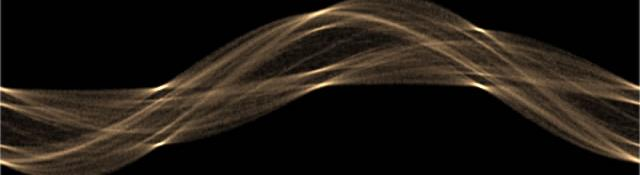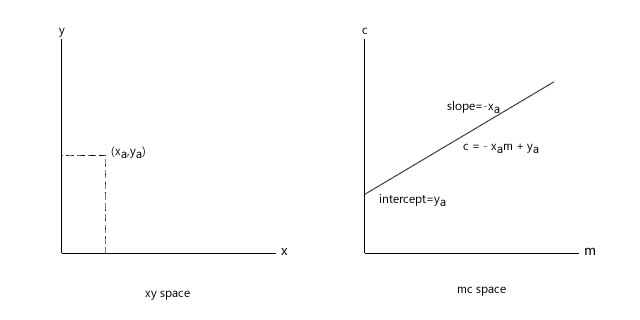## The Basics

Series: The Hough Transform:

1. The Basics
2. The Normal form

The Hough transform is an incredible tool that lets you identify lines. Not just lines, but other shapes as well. In this article, I'll talk about the mechanics behind the Hough transform. It will involve a bit of math, but just elementary concepts you learned in school. In this article, we'll work with lines only, though the technique can be easily extended to other shapes.

## Why the Hough Transform?

Lets say you take the snapshot of pole. You figure out edge pixels (using the Canny edge detector, the Sobel edge detector, or any other thing). Now you want a geometrical representation of the pole's edge.You want to know its slope, its intercept, etc. But right now the "edge" is just a sequence of pixels.

You can loop through all pixels, and some how figure out the slope and intercept. But that is one difficult task. Images are never perfect.

So you want some mechanism that give more weightage to pixels that are already in a line. This is exactly what the Hough Transform does.

It lets each point on the image "vote". And because of the mathematical properties of the transform, this "voting" allows us to figure out prominent lines in the image.

## From lines to points

A lines is a collection of points. And managing a collection of points is tougher than managing a single point. Obviously. So the first thing we learn is how to represent a line as a single point, without losing any information about it.

This is done through the m-c space.

As shown in the above picture, every line has two quantities associated with it, the slope and the intercept. With these two numbers, you can describe a line completely.

So the parameter space, or the mc space was devised. Every line in the xy space is equivalent to a single point in the mc space. Neat eh?

## From points to lines

Now onto the next step. Consider a point (say, (xa, ya) )in the xy space. What would its representation in the mc space be?

For starters, you could guess that infinite lines pass through a point. So, for every line passing through (xa, ya), there would be a point in the mc space.

And you're correct there, because of the following:

1. Any line passing through (xa, ya): ya = mxa + c
2. Rearranging: c = - xam + ya
3. The above is the equation of a line in the mc space.

So, a point in the xy space is equivalent to a line in the mc space.## How does this help us?

The Hough transform is all about doing what we just learned: converting points in the xy space to lines in the mc space.

You taken an edge detected image, and for every point that is non black, you draw lines in the mc place. Obviously, some lines will intersect. These intersections mark are the parameters of the line.

The following picture will clarify the idea:

The points 1, 2, 3, and 4 are represented as various lines in the mc space. And the intersection of these lines is equivalent to the original line.

## Great!

Now you know the basic idea of how the Hough transform works. But there's one big flaw in the We'll discuss how to resolve this issue in the next article.

## More in the series

This tutorial is part of a series called The Hough Transform:

1. The Basics
2. The Normal form

#### Related posts

Utkarsh Sinha created AI Shack in 2010 and has since been working on computer vision and related fields. He is currently at Microsoft working on computer vision.